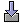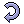# Visualisation for the Tableau calculus

The Tableau Calculus is a formal method to prove statements in First Order Predicate Logic. It is explained in extenso for instance in the scriptum to the lecture formal methods (German).

2007-2016 by Mattias Ulbrich

## Using the applet

A new proof can be started using the-Button. You will be asked to enter one or more formulas spearated by ';'. See description below on how to enter formulas.

Nodes in the proof are color-coded according to their "type" of formula:

 ALPHA Non-branching formulas with subformulas (such as A ∧ B) BETA Branching formulas with subformulas (such as A ∨ B) GAMMA Universally quantified formula (∀x. A(x)) DELTA Existentially quantified formula (∃x. A(x)) NEGNEG Special case: Double negation (¬¬A), resolves to A

You can drag a formula holding your left mouse button (formula becomes red) onto a leaf of the tree to apply the according rule to this branch. The leaf must not be closed. If you want to expand a formula on the spot, you can click on it twice. You can also drag a formula with your right mouse button pressed (formula becomes green) onto a contradictory formula (i.e. A onto ¬ A or v.v.) to close branches.

The application of gamma rules create new free variables called `Xn` with n a natural number. These free variables can be instantiated using the-button. A message box pops up and you can enter the instantiation for one free variable in the form `Xn = formula`.

You can undo rule applications and instantiations using the-button. Please note that closed branches may become reopened hereby.

If the formulas are not clearly visible or if you prefer ASCII rather than mathematical formulas, you can switch between ASCII and Unicode characters using the-button.

## On the notation of formulas

The grammar for formulas is straight forward and will be presented here by examples. Identifiers can be any character string (case sensitive!) starting with a letter (optionally) followed by letters or digits. You must not use `X1, X2, ...` and `sk1, sk2, ...` however, for these are reserved for free variables and skolem symbols respectively.

 `A x. p(x)` for all x, p(x) holds. p is a predicate here. `E x. p(f(x))` There is a x so that p(f(x)) holds. p is a predicate and f is a function here `P & Q` P and Q hold. `P -> Q` P implies Q `P | Q` P or Q `P | Q & R` Operators have the usual precedence: & then | then ->. I.e. `P | Q & R = P | (Q & R)` `A x. p(x) | q(x)` Caution! This is equal to `(A x. p(x)) | q(x)`. Therefore x appears as free variable which may not be. The applet does not check for such things, so be aware!

## Applet

This allows you to run the program in your browser. It may be that you have disabled Java applets. Either activate them or choose another method of running the program from the top of this page.